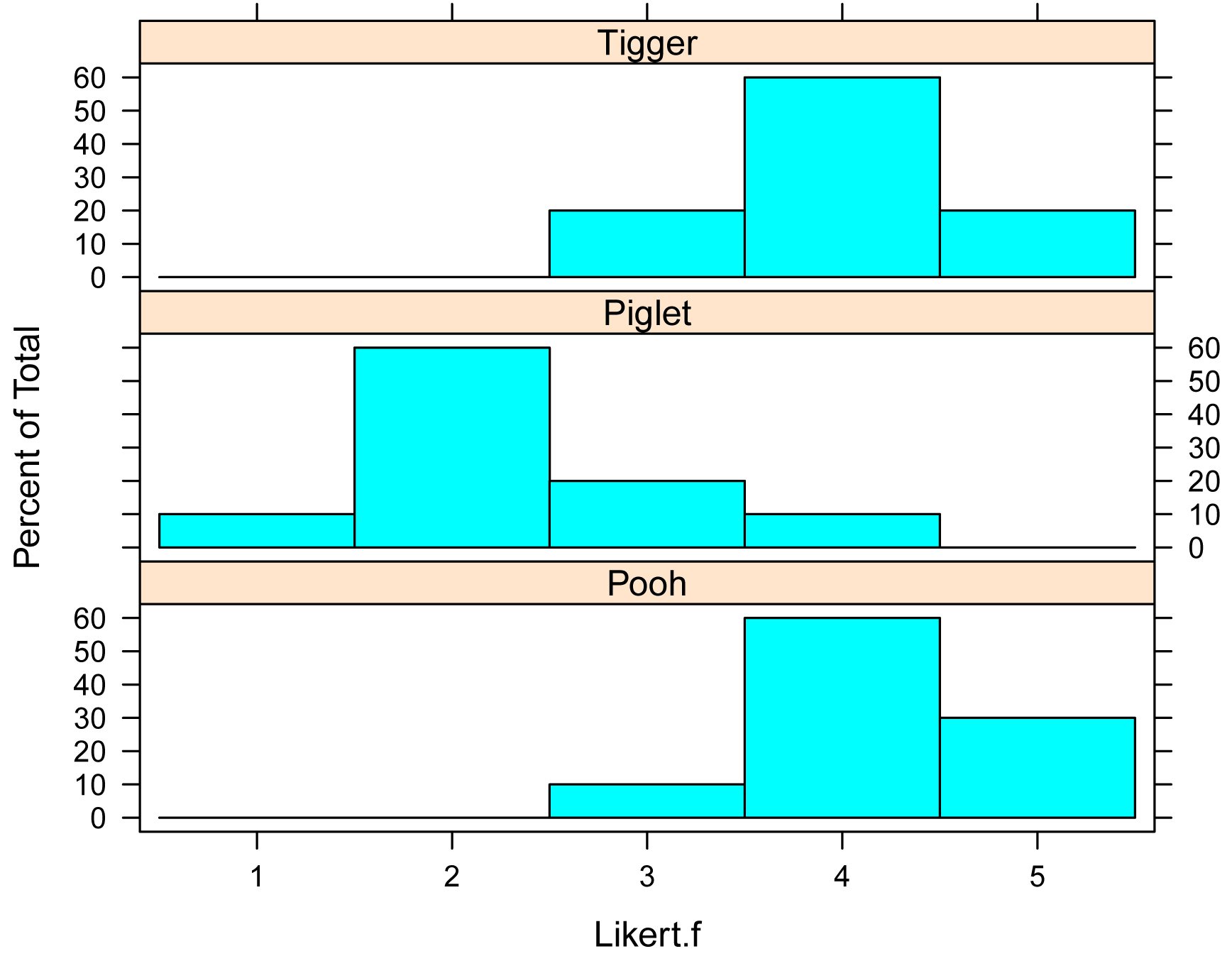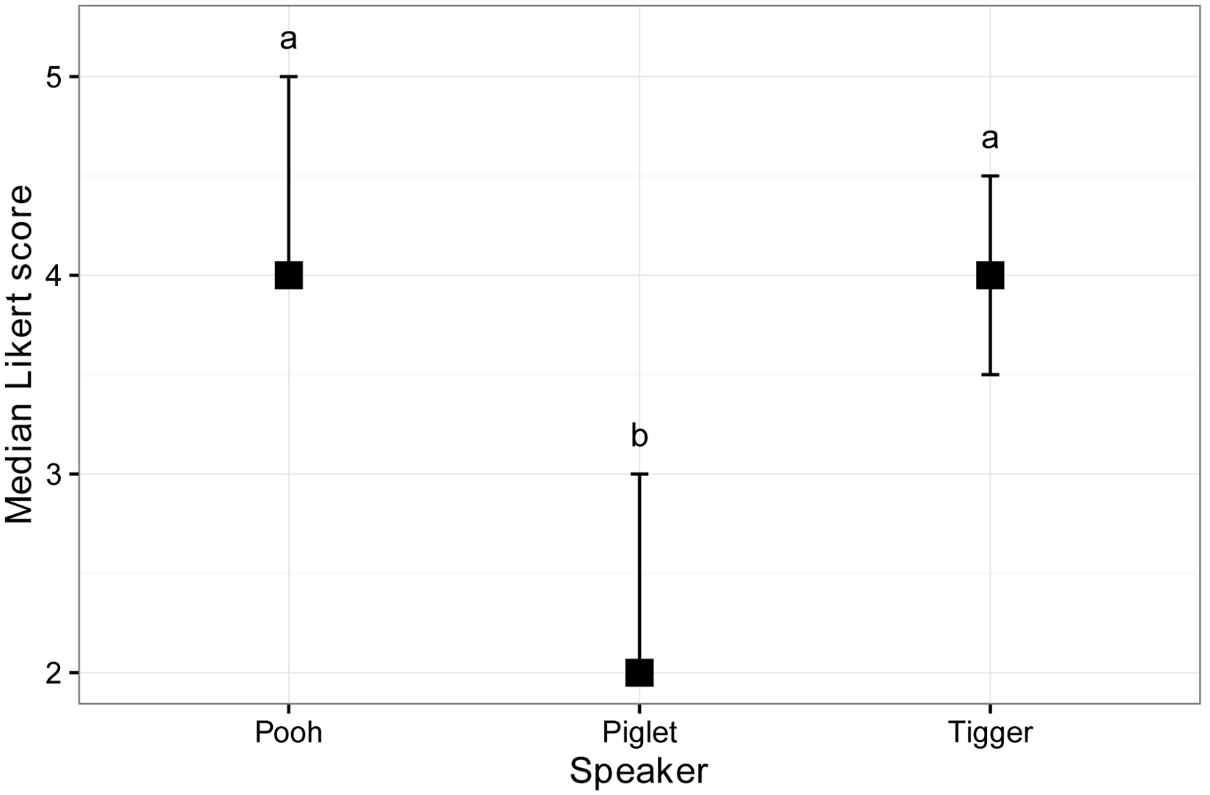## Summary and Analysis of Extension Program Evaluation in R

Salvatore S. Mangiafico

# One-way Permutation Test of Independence for Ordinal Data

### When to use this test

A permutation test of independence can be used for one-way data with an ordinal dependent variable.  It will determine if there is a difference in the response variable among groups.  There can be two or more groups.

This test covers the designs used with Mann–Whitney, Kruskal–Wallis, two-sample t-test, one-way anova, one-way anova with blocks, and ordinal regression equivalents.

The test assumes that the observations are independent.  That is, it is not appropriate for paired observations or repeated measures data.  It does not make assumptions about the distribution of values.

The test is performed with the independence_test function in the coin package.

Post-hoc testing can be conducted with pairwise permutation tests across groups.

It is important that the dependent variable be specified as an ordered factor variable in R.

##### Appropriate data

•  One-way data.  That is, one measurement variable in two or more groups

•  Dependent variable is ordinal, and specified in R as an ordered factor variable

•  Independent variable is a factor with two or more levels.  That is, two or more groups

•  Observations between groups are independent.  That is, not paired or repeated measures data

##### Hypotheses

•  Null hypothesis:  The values of the dependent variable among groups are equal.

•  Alternative hypothesis (two-sided): The values of the dependent variable among groups are not equal.

##### Interpretation

•  Reporting significant results for the omnibus test as “Significant differences were found among values for groups.” is acceptable.  Alternatively, “A significant effect for Independent Variable on Dependent Variable was found.”

•  Reporting significant results for mean separation post-hoc tests as “Value of Dependent Variable for group A was different than that for group B.” is acceptable.

##### Other notes and alternative tests

Ordinal regression is an alternative.

The traditional nonparametric tests Mann–Whitney U or Kruskal–Wallis are alternatives in cases where there is not a blocking variable.

### Packages used in this chapter

The packages used in this chapter include:

•  psych

•  lattice

•  FSA

•  coin

•  rcompanion

•  multcompView

•  ggplot2

The following commands will install these packages if they are not already installed:

if(!require(psych)){install.packages("psych")}
if(!require(FSA)){install.packages("FSA")}
if(!require(lattice)){install.packages("lattice")}
if(!require(coin)){install.packages("coin")}
if(!require(rcompanion)){install.packages("rcompanion")}
if(!require(multcompView)){install.packages("multcompView")}
if(!require(ggplot2)){install.packages("ggplot2")}

### One-way ordinal permutation test example

This example re-visits the Pooh, Piglet, and Tigger data from the Descriptive Statistics with the likert Package chapter.

It answers the question, “Are the scores significantly different among the three speakers?”

Note that a new variable, Likert.f, is created for the Likert scores as an ordered factor variable.  It is necessary that the dependent variable passed to independence_test be an ordered factor variable for it to be treated as an ordinal variable.

Input =("
Speaker  Likert
Pooh      3
Pooh      5
Pooh      4
Pooh      4
Pooh      4
Pooh      4
Pooh      4
Pooh      4
Pooh      5
Pooh      5
Piglet    2
Piglet    4
Piglet    2
Piglet    2
Piglet    1
Piglet    2
Piglet    3
Piglet    2
Piglet    2
Piglet    3
Tigger    4
Tigger    4
Tigger    4
Tigger    4
Tigger    5
Tigger    3
Tigger    5
Tigger    4
Tigger    4
Tigger    3
")

### Order levels of the factor; otherwise R will alphabetize them

Data\$Speaker = factor(Data\$Speaker,
levels=unique(Data\$Speaker))

### Create a new variable which is the likert scores as an ordered factor

Data\$Likert.f = factor(Data\$Likert,
ordered = TRUE)

###  Check the data frame

library(psych)

str(Data)

summary(Data)

### Remove unnecessary objects

rm(Input)

#### Summarize data treating Likert scores as factors

Note that the variable we want to count is Likert.f, which is a factor variable.  Counts for Likert.f are cross tabulated over values of Speaker.  The prop.table function translates a table into proportions.  The margin=1 option indicates that the proportions are calculated for each row.

xtabs( ~ Speaker + Likert.f,
data = Data)

Likert.f
Speaker  1 2 3 4 5
Pooh   0 0 1 6 3
Piglet 1 6 2 1 0
Tigger 0 0 2 6 2

XT = xtabs( ~ Speaker + Likert.f,
data = Data)

prop.table(XT,
margin = 1)

Likert.f
Speaker    1   2   3   4   5
Pooh   0.0 0.0 0.1 0.6 0.3
Piglet 0.1 0.6 0.2 0.1 0.0
Tigger 0.0 0.0 0.2 0.6 0.2

#### Bar plots by group

Note that the variable we want to count is Likert.f, which is a factor variable.  Counts for Likert.f are presented for values of Speaker.

library(lattice)

histogram(~ Likert.f | Speaker,
data=Data,
layout=c(1,3))      #  columns and rows of individual plots#### Summarize data treating Likert scores as numeric

It may be useful to look at the minimum, first quartile, median, third quartile, and maximum for Likert for each group.

library(FSA)

Summarize(Likert ~ Speaker,
data=Data,
digits=3)

Speaker  n mean    sd min Q1 median   Q3 max percZero
1    Pooh 10  4.2 0.632   3  4      4 4.75   5        0
2  Piglet 10  2.3 0.823   1  2      2 2.75   4        0
3  Tigger 10  4.0 0.667   3  4      4 4.00   5        0

#### One-way ordinal permutation test

Note that the dependent variable is an ordered factor variable, Likert.fSpeaker is the independent variable.  The data= option indicates the data frame that contains the variables.  For the meaning of other options, see library(coin); ?independence_test. There is the option of adding a blocking variable to the formula with e.g. | Block.

library(coin)

independence_test(Likert.f ~ Speaker,
data = Data)

Asymptotic General Independence Test

maxT = 4.2006, p-value = 7.685e-05

#### Post-hoc test: pairwise permutation tests

If the independence test is significant, a post-hoc analysis can be performed to determine which groups differ from which other groups.

The pairwisePermutationTest and pairwisePermutationMatrix functions in the rcompanion package conduct permutation tests across groups in a pairwise manner.   See library(rcompanion); ?pairwisePermutationTest for further details.

Because the post-hoc test will produce multiple p-values, adjustments to the p-values can be made to avoid inflating the possibility of making a type-I error.  Here, the method of adjustment is indicated with the method option.  There are a variety of methods for controlling the familywise error rate or for controlling the false discovery rate.  See ?p.adjust for details on these methods.

Before conducting the pairwise tests, we will re-order the levels of the grouping variable by the median of each group.  This makes interpretation of the pairwise comparisons and compact letter display easier.  In the output, groups sharing a same letter are not significantly different.

### Order groups by median

Data\$Speaker = factor(Data\$Speaker,
levels=c("Pooh", "Tigger", "Piglet"))

### Pairwise permutation tests

library(rcompanion)

PT = pairwisePermutationTest(Likert.f ~ Speaker,
data   = Data,
method = "fdr")

PT

1   Pooh - Tigger = 0  0.698    0.4852 0.485200
2   Pooh - Piglet = 0  3.515  0.000439 0.001238
3 Tigger - Piglet = 0 -3.344 0.0008254 0.001238

### Compact letter display

library(rcompanion)

data = PT,
threshold  = 0.05)

Group Letter MonoLetter
1   Pooh      a         a
2 Tigger      a         a
3 Piglet      b          b

Groups sharing a letter are not significantly different (alpha = 0.05).

##### Matrix output and compact letter display

The code creates a matrix of p-values called PM, which is then passed to the multcompLetters function to be converted to a compact letter display.

Here the fdr p-value adjustment method is used.  See ?p.adjust for details on available methods.

### Order groups by median

Data\$Speaker = factor(Data\$Speaker,
levels=c("Pooh", "Tigger", "Piglet"))

### Conduct pairwise permutation tests

library(rcompanion)

PM = pairwisePermutationMatrix(Likert.f ~ Speaker,
data   = Data,
PM

### Produce compact letter display

library(multcompView)

multcompLetters(PM,
compare="<",
threshold=0.05,  # p-value to use as significance threshold
Letters=letters,
reversed = FALSE)

Pooh Tigger Piglet
"a"    "a"    "b"

Groups sharing a letter are not significantly different (alpha = 0.05).

#### Plot of medians and confidence intervals

The following code uses the groupwiseMedian function to produce a data frame of medians for each speaker along with the 95% confidence intervals for each median with the percentile method.  See library(rcompanion); ?groupwiseMedian for further options.

These medians are then plotted, with their confidence intervals shown as error bars.  The grouping letters from the multiple comparisons are added.

### Order groups by original order

Data\$Speaker = factor(Data\$Speaker,
levels=c("Pooh", "Piglet", "Tigger"))

### Create data frame of medians and confidence intervals

library(rcompanion)

Sum = groupwiseMedian(Likert ~ Speaker,
data       = Data,
conf       = 0.95,
R          = 5000,
percentile = TRUE,
bca        = FALSE,
digits     = 3)

Sum

### Prepare labels

X = 1:3

Y = Sum\$Percentile.upper + 0.2

Label = c("a", "b", "a")

### Plot

library(ggplot2)

ggplot(Sum,                ### The data frame to use.
aes(x = Speaker,
y = Median)) +
geom_errorbar(aes(ymin = Percentile.lower,
ymax = Percentile.upper),
width = 0.05,
size  = 0.5) +
geom_point(shape = 15,
size  = 4) +
theme_bw() +
theme(axis.title   = element_text(face  = "bold")) +

ylab("Median Likert score") +

annotate("text",
x = X,
y = Y,
label = Label)Plot of median Likert score versus Speaker.  Error bars indicate the 95% confidence intervals for the median with the percentile method.

### Comparison of methods for independence tests for ordinal data

Data                Test                p-value   Mean separation
Pooh Piglet         Permutation         0.0004
Pooh Piglet         Ordinal regression  0.00003
Pooh Piglet         Mann–Whitney        0.0005

Pooh Tigger Piglet  Permutation         0.00008   a  a  b
Pooh Tigger Piglet  Ordinal regression  0.000008  a  a  b
Pooh Tigger Piglet  Kruskal–Wallis      0.0002    a  a  b